# Calculating Ideal Spring and Roll Bar Rates Part 2Accounting for Tyres

So far in the process we have ignored the effects of tyres for calculating our spring rates.

One aspect of the tyre to consider is the grip generated due to the normal force being applied through the tyre. This is the grip generated due to the mass acting upon the wheel and the friction co-efficient of the compound of the tyre. Earlier in the process in part 1 we calculated the lateral load transfer onto the outside wheels and generated a value for the mass present at the outside and inside wheels in the mid corner section of the circuit. These values were:

• Front Outside Wheel = 686.6 kg
• Front Inside Wheel = 233.4 kg
• Rear Outside Wheel = 471.87 kg
• Rear Inside Wheel = 148.13 kg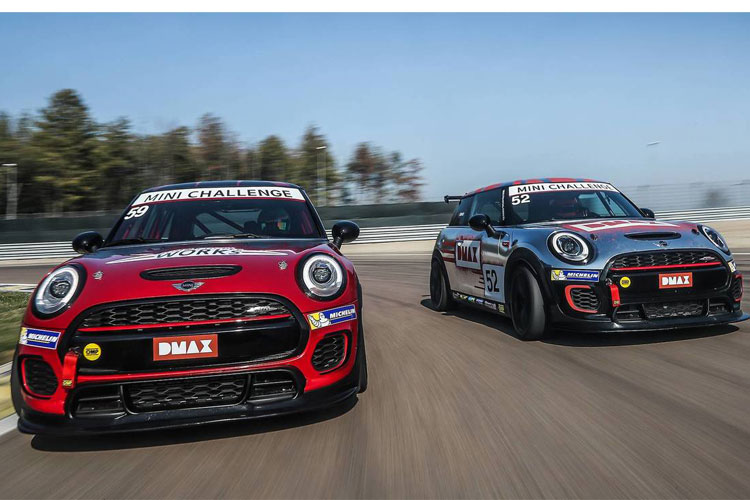If you are racing in a championship where only one tyre is allowed then it would be helpful to input this tyre data at this stage to increase the accuracy of the calculations for your application. You should be able to obtain a normal force vs lateral traction force graph for your tyres either directly from the tyre manufacturer or from their website. This graph will tell you how much lateral force each loaded tyre is capable of generating. This will tell us if the front and rear tyres are capable of handling the cornering force that the car is experiencing in this cornering situation. In our example the cornering force experienced is:

• Lateral Acceleration: 1.13G

However, we need this in newtons so will use the conversion of:

• 1G = 9.81m/s²

Therefore our lateral acceleration experienced is:

• Lateral Acceleration: 11.0853 m/s²

Now we can use this acceleration figure to calculate our centripetal force acting upon the car as a result of the lateral acceleration and the vehicle mass using the equation:Therefore our centripetal force is: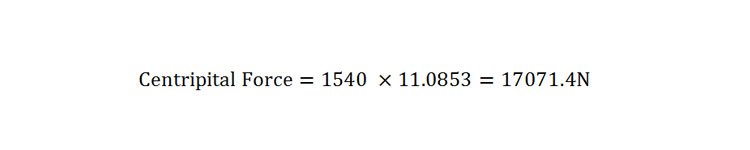Next we need to calculate the load on each tyre during the cornering situation and convert our values of mass to values of force using the conversion of (1kg = 9.81N). Therefore the load on each wheel during the cornering situation is:

• Front Outside Wheel = 686.6 kg = 6735N
• Front Inside Wheel = 233.4 kg = 2289.7N
• Rear Outside Wheel = 471.87 kg = 4629N
• Rear Inside Wheel = 148.13 kg = 1453.15N

The graph below shows a normal force vs lateral traction force graph for an example tyre on our car.During cornering all 4 tyres will oppose the lateral acceleration force so will share the load. Using the above diagram for the tyres we can observe the graph to see how much lateral traction force the tyre can generate with the normal force acting upon the tyre in the cornering situation. Looking at the graph you can see that each tyre has the following lateral traction forces:

• Front Outside Wheel = 7400N
• Front Inside Wheel = 2700N
• Rear Outside Wheel = 5600N
• Rear Inside Wheel = 1800N

We can now add all of these lateral traction forces together to produce our overall lateral traction force that the tyres can generate combined:

• Total Lateral Traction Force = 17500N

As our centripetal force acting upon the chassis was a lower value than our combined lateral traction force from the tyres this tells us that the car will maintain grip around this corner so we are fine to continue with the process. If the values for your tyres were too low meaning that in this scenario your car will lose traction then there are a few options:

• Change your tyre choice to a more grippy compound with a graph that works
• Alter roll rates earlier in the process to reduce the lateral load transfer rate
• Reduce the mass of your car
• Lower roll centre heights of the affected axle
• Reduce Centre of gravity height
• Go through the corner slower

Whichever decision is made from the above options you will have to go back to the start of the calculations in part 1 to alter the chosen figure which will alter the answers throughout the process so far. The only option that doesn’t require this is changing tyre choice.

Calculating Wheel Centre RatesWheel Centre Rates for Independent Suspension

With the tyre selected able to generate our required cornering forces we can add in our tyre vertical spring rate to come to a wheel centre rate. In reality there are two springs acting between the chassis and the ground. These two springs are the wheel centre rate, controlled by the spring and anti-roll bar, and the vertical tyre rate, controlled by the tyre compound, sidewall stiffness and operating pressure.

In order to combine these two spring effects to create one we must add them together. Although they are acting in series, the equation is slightly different than our linear springs in series equation due to the tyre rate being involved. Therefore, we use the similar but different equation below to calculate the wheel rate:Where:

• Kw = Wheel Centre Rate (N/m)
• Kr = Ride Rate (N/m)
• Kt = Tyre Vertical Rate (N/m)

Tyre vertical rates are often given by tyre manufactures in kg/mm. To convert from this to “N/m” for use within the equations, use the conversion factor below. Our example tyre has a vertical stiffness of 35 kg/mm.With our tyre vertical spring rate we can now calculate our front and rear wheel centre rates.And:When a tyre rate is included in the equation the wheel centre rate often becomes higher than the ride rate. With the new wheel centre rates now known using the ride rates we can estimate the anti-roll bar spring rate required for the system using the following equation:Where:

• KѲA = Required Anti-Roll Bar Rate (Nm/radian)
• KѲ = Assumed Initial Roll Rate (Nm/radian)
• Kw = Wheel Centre Rate (N/m)
• t = Track Width (m)
• Kt = Tyre Rate (N/m)

Using all the above figures that we have previously calculated we can carry out the equation as follows for the front and rear roll bars using front and rear wheel centre rates, track widths and assumed roll rates respectively.And: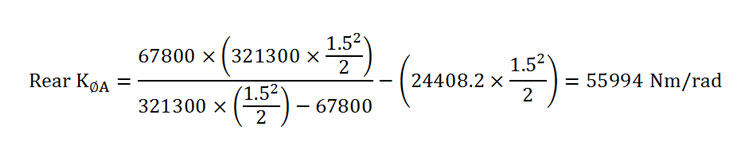Therefore:

Front Anti-roll bar rate = 89351 Nm/rad

Rear Anti-roll bar rate = 55994 Nm/rad

As you can see, with our tyre vertical rate accounted for, the required anti-roll bar spring rate has increased substantially from our first attempt at calculating required roll bar rates.

Calculating Rates for An Axle SuspensionBeam axle suspension has a slightly more complex approach to calculating the ride and roll rates due to springs not being located at the outboard track locations like an independent suspension system. Due to the springs being further inboard of the track, the spring rates are effectively softer in roll than the equivalent rates would be in an independent suspension system. Therefore the springs must be decoupled from ride and roll modes and we must look directly at the springs in their mounted position at the axle as oppose to using ideal ride and roll rates.

Some of the same equations from the above sections will be used throughout and some in a similar form. First we must calculate the vertical axle rate of the suspension. This is similar to calculating the wheel rate of an independent suspension when accounting for the vertical tyre spring rate. The vertical axle rate is the installed spring rate for a leaf spring suspended axle.  The equation below can be used to find the vertical axle spring rate:Where:

• Kw = Vertical Axle Rate (N/m)
• Kr = Ride Rate (N/m)
• Kt = Tyre Rate (N/m)

With the vertical axle rate calculated we can now find the roll rate due to the vertical axle rate using the below equation:Where:

• Kt = Tyre Rate (N/m)
• Kw = Vertical Tyre Axle Rate (N/m)
• Tr = Rear Track Width (m)
• Ts = Spring Track Width (m)

If the rear axle has an anti-roll bar mounted to it then the equation becomes even more complex to calculate the roll rate. The below equation should be used if an anti-roll bar is mounted to the rear beam axle.Where:

• Rarb = Roll Rate of the Anti-Roll Bar (Nm/rad of body roll)
• Kt = Tyre Rate (N/m)
• Kw = Vertical Tyre Axle Rate (N/m)
• Tr = Rear Track Width (m)
• Ts = Spring Track Width (m)

Due to the lateral and torsional deflection caused by leaf springs when in roll, they produce a higher roll rate than that of the vertical axle rate. The roll rate is typically between 30-50% greater than the vertical axle rate. For this example we will use the figure of a 40% increase. Therefore we can now calculate the combined roll rate of the springs and the roll bar.We can now insert some values into the above equations to determine revised weight transfers and roll gradients if a beam axle is installed in the rear of your car. If we take our previous example figures for our independently suspended car and alter it to have a rear live axle suspended by leaf springs with a spring track width of 1 meter but with the same rear ride rates as calculated earlier then the vertical axle rate becomes:Then the roll rate can be calculated using:Therefore the rear roll rate becomes:As we expected this is a lot less stiff in roll than an independently suspended system as with our independent suspension we got 25520.5 Nm/radian which is considerably higher than this value. This is due to the leaf springs being mounted much further inboard of the wheel centres than independent suspension. With the roll rate we can now input this into our roll gradient equation to generate a new one for the beam axle. Due to there being no anti-roll bar on the back of the leaf spring rear end example the rear roll figures are altered from the independent example.Which produces new front and rear weight transfers, assuming that the roll centre positions are not altered, of:And:And our outside wheel loads are:

• Front Outside Wheel = W(fo) = W1+Wf = 460+331.6 = 791.6 kg
• Rear Outside Wheel = W(ro) = W3 + Wr = 310+56.9 = 366.9 kg

And our changes from static wheel loadings for our outside wheels are:

• Front Outside Wheel Change=ΔW(fo) = W(fo)-W1 =791.6 – 460= +331.6 kg
• Rear Outside Wheel Change = ΔW(ro) = W(ro)-W3 = 366.9 – 310= +56.9 kg

Due to the lack of a rear roll bar and softer rear roll stiffness in general you can see a much lower load transfer across the rear wheels and a slightly higher front load transfer compared to our independent suspension example from earlier.

Final Stages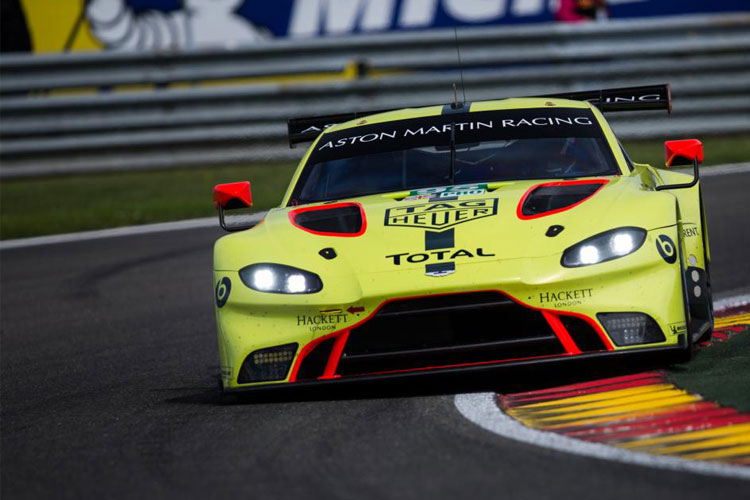Returning to our independent suspension example from earlier we can pick up where we left off with our ideal ride and roll rates with the vertical tyre spring force accounted for. So far we have calculated our ideal wheel rate and our ideal roll rate all referred at the wheel. We must now use installation ratios and anti-roll bar contribution rate to calculate the actual spring rate of the part that will be bolted to the car in the form of coil springs and anti-roll bars.

For the anti-roll bar we need to know the installation ratio in terms of anti-roll bar twist per degree of chassis roll. For the equation of the contribution of the anti-roll bar we need to know:

• The installation ratio of the anti-roll bar covered in our “Installation Ratio” article. This is the ratio regarding where the drop link mounts to the arm, the distance to the wheel centre and the overall length of the arm.
• The anti-roll bar cantilever length, measured from the centre line of the roll bar to where the canti-lever mounts to the drop link.

The equation for the contribution of the anti-roll bar is:Where:

• KθA = Anti-Roll Bar Rate Contribution to Car Roll Rate (Nm/radian)
• KθB = Anti-Roll Bar Angular Twist Rate (Nm/radian)
• L = Anti-Roll Bar Cantilever Length (m)
• Ib = Installation Ratio of the Anti-Roll Bar
• T = Track Width (m)

Now we can calculate our spring and anti-roll bar rates. You will need the installation ratio of your front and rear springs as obtained from the “installation Ratio” article. With these we can calculate our front and rear spring rates using our ideal front and rear ride rates referred at the wheel from earlier with the below equation:And:Where:

• Ksf = Front Spring Rate (N/m)
• Ksr = Rear Spring Rate (N/m)
• Kwf = Front Ride Wheel Rate (N/m)
• Kwr = Rear Ride Wheel Rate (N/m)
• IRf = Installation Ratio of Front Spring
• IRr = Installation Ratio of Rear Spring

And re-arranging the above anti-roll bar contribution equation to produce the anti-roll bar angular twist rate we can calculate our front and rear anti-roll bar stiffness’s using:And:Where:

• Kθaf = Front Anti-Roll Bar Rate Contribution to Car Roll Rate (Nm/radian)
• Kθf = Front Anti-Roll Bar Angular Twist Rate (Nm/radian)
• Lf = Front Anti-Roll Bar Cantilever Length (m)
• Ibf = Front Installation Ratio of the Anti-Roll Bar
• Tf = Front Track Width (m)
• Kθar =Rear Anti-Roll Bar Rate Contribution to Car Roll Rate (Nm/radian)
• Kθr = Rear Anti-Roll Bar Angular Twist Rate (Nm/radian)
• Lr = Rear Anti-Roll Bar Cantilever Length (m)
• Ibr = Rear Installation Ratio of the Anti-Roll Bar
• Tr = Rear Track Width (m)Now we can insert all of our previous results into the above four equations to produce our front and rear anti-roll bar and spring rates:

Spring RatesAnd: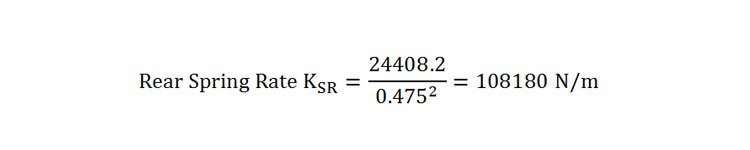Anti-Roll Bar Rates:And:These values can now be used to find actual springs and anti-roll bars that fall close to these figures as it is unlikely to get exactly what your result is unless they are being custom made for your vehicle. Also, for the anti-roll bar stiffness values, select a roll bar that has adjustable stiffness and try to get one where your calculated stiffness is the centre point of adjustment. This will help with tuning the set up once installed as this is a base set-up.

Finally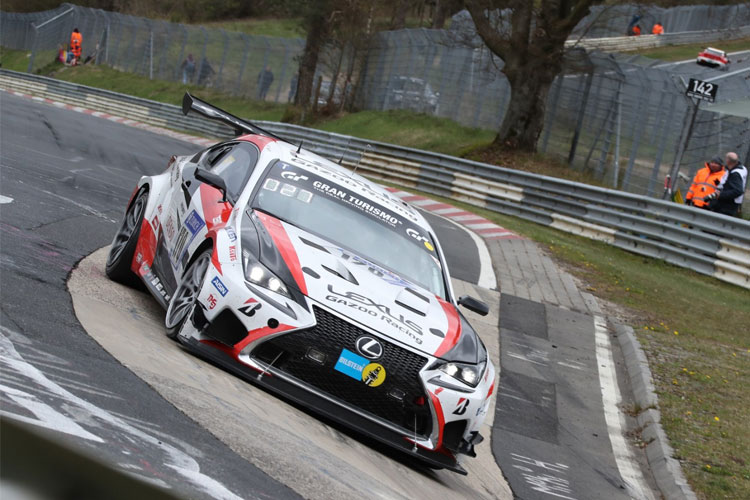We must close these calculations with a note that all of the above examples are based upon an ideal car where there is no chassis flex in torsion, all joints are totally free of friction and that there is no movement in any mounting points or fixings etc. Therefore, these figures are a good starting point to install spring rates on your race car to begin with but we advise testing the car with these rates that they might have to alter slightly for your application to fine tune the vehicle to your preferences of handling.

Ref: Equations derived from: Milliken, W. and Milliken, D., 1995. Race Car Vehicle Dynamics. 1st ed. United States of America: SAE Publications Group.

### 8 thoughts on “Calculating Ideal Spring and Roll Bar Rates Part 2”

1.Nora Enderby says:

Hi! I am a member of a formula student team and I was wondering about the ARB calculations. It seems like you have followed the Milliken books formulas in chapter “Ride and roll rates – 16.2 first example with more complete calculations”. Do you have a metric version of “16.5 Second example with simplified calculations” as well?

Thanks, Nora Enderby

2.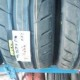Mohamed Anwar says:

How can I factor in the anti-squat and anti-dive geometry: just decrease the final spring rates by the anti’s % linearly? Thanks

1.Suspension Secrets says:

Hi,

The calculation process is to give you ideal spring rates to go testing on. You should follow the steps disregarding anti’s then go testing with that set up and tweak from there as the amount of anti’s required will alter with a brand new spring set up.

1.Mohamed Anwar says:

Thanks a lot!

3.Fabrizio Torri says:

Isn’t the formula to calculate springs in series (K1*K2)/(K1+K2)?

1.Suspension Secrets says:

HI Fabrizio, yes it is. However, due to the tyre rate being included, the equation for wheel centre rate is slightly different to that of the springs in series equation.

Thanks

1.Fabrizio Torri says:

So what if K1 = K2? You’ll find yourself with a division by 0… isn’t it?